# We Need to Talk Videos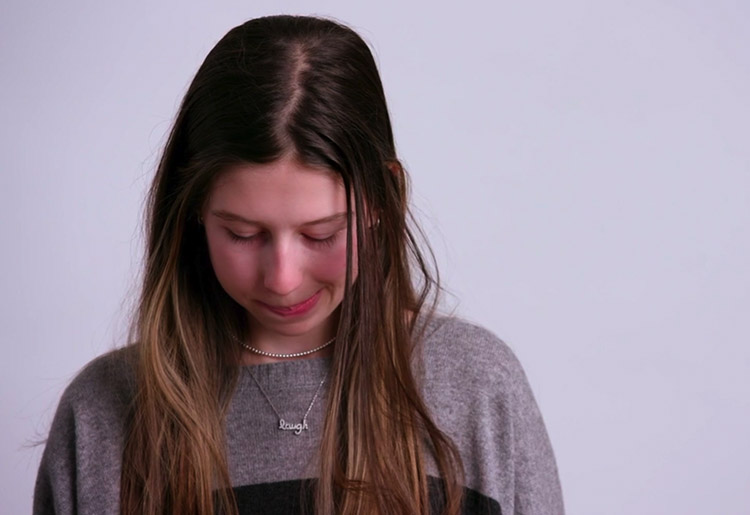### One Thing I Wish You Knew 2019 /* <![CDATA[ */ jQuery(document).ready(function(\$){ \$(function(){ var width = \$(window).innerWidth(); var setwidth = 960; var ratio = 0.5625; var height = 540; var link = 'https://vimeo.com/324983095?width=960&height=540'; if(width < setwidth) { height = Math.floor(width * 0.5625); //console.log("device width "+width+", set width "+960+", ratio "+0.5625+", new height "+ height); var new_url = wpvl_paramReplace('width', link, width); var new_url = wpvl_paramReplace('height', new_url, height); \$("a#5c94f3ca3bb38").attr('href', new_url); //console.log(new_url); } }); }); /* ]]&gt; */

#### watch the video /* <![CDATA[ */ jQuery(document).ready(function(\$){ \$(function(){ var width = \$(window).innerWidth(); var setwidth = 960; var ratio = 0.5625; var height = 540; var link = 'https://vimeo.com/324983095?width=960&height=540'; if(width < setwidth) { height = Math.floor(width * 0.5625); //console.log("device width "+width+", set width "+960+", ratio "+0.5625+", new height "+ height); var new_url = wpvl_paramReplace('width', link, width); var new_url = wpvl_paramReplace('height', new_url, height); \$("a#5c94f3ca3bb58").attr('href', new_url); //console.log(new_url); } }); }); /* ]]&gt; */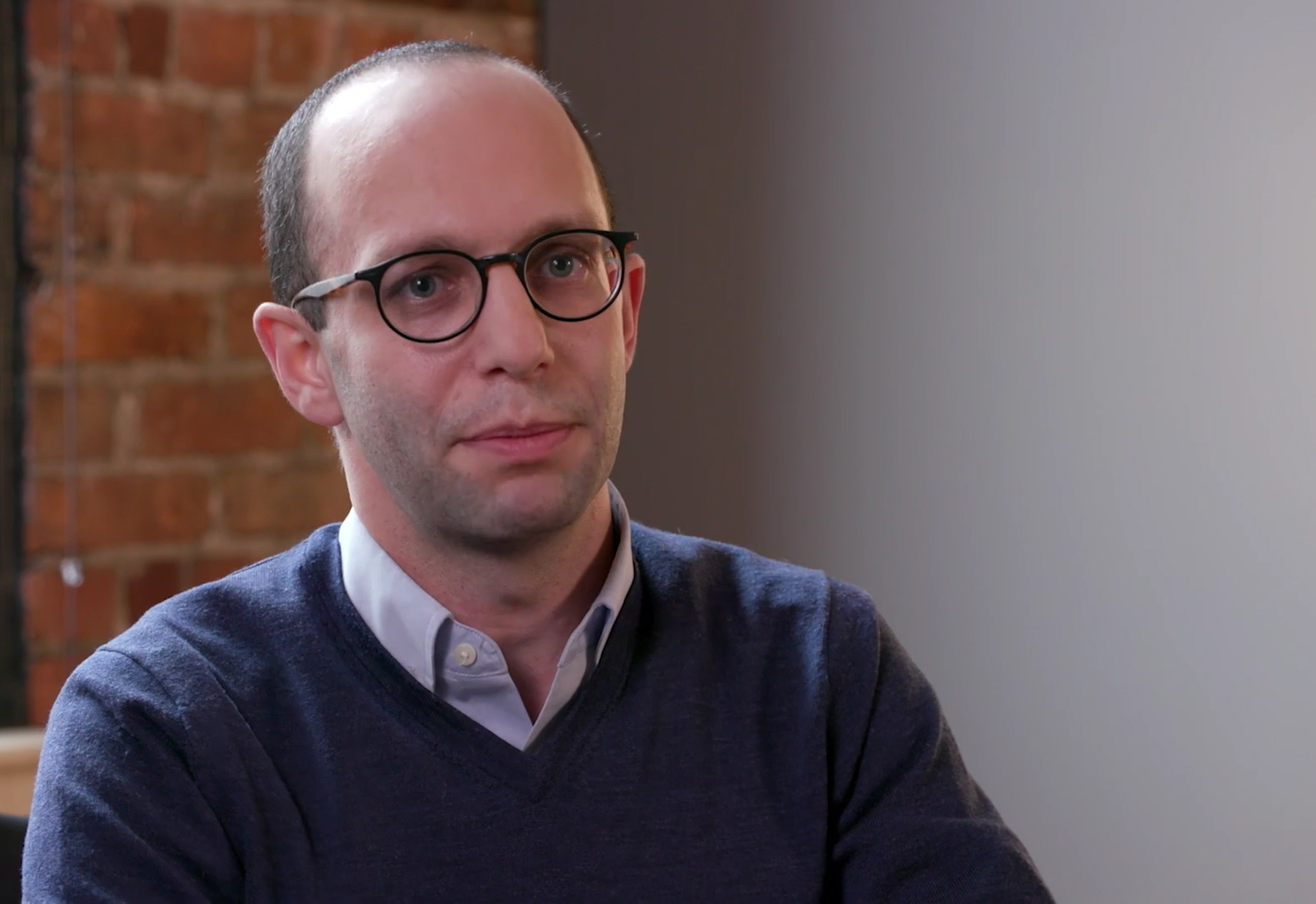### What are depression and anxiety? /* <![CDATA[ */ jQuery(document).ready(function(\$){ \$(function(){ var width = \$(window).innerWidth(); var setwidth = 960; var ratio = 0.5625; var height = 540; var link = 'https://vimeo.com/308560633?width=960&height=540'; if(width < setwidth) { height = Math.floor(width * 0.5625); //console.log("device width "+width+", set width "+960+", ratio "+0.5625+", new height "+ height); var new_url = wpvl_paramReplace('width', link, width); var new_url = wpvl_paramReplace('height', new_url, height); \$("a#5c94f3ca3bb97").attr('href', new_url); //console.log(new_url); } }); }); /* ]]&gt; */

#### watch Jeremy's video /* <![CDATA[ */ jQuery(document).ready(function(\$){ \$(function(){ var width = \$(window).innerWidth(); var setwidth = 960; var ratio = 0.5625; var height = 540; var link = 'https://vimeo.com/308560633?width=960&height=540'; if(width < setwidth) { height = Math.floor(width * 0.5625); //console.log("device width "+width+", set width "+960+", ratio "+0.5625+", new height "+ height); var new_url = wpvl_paramReplace('width', link, width); var new_url = wpvl_paramReplace('height', new_url, height); \$("a#5c94f3ca3bbb5").attr('href', new_url); //console.log(new_url); } }); }); /* ]]&gt; */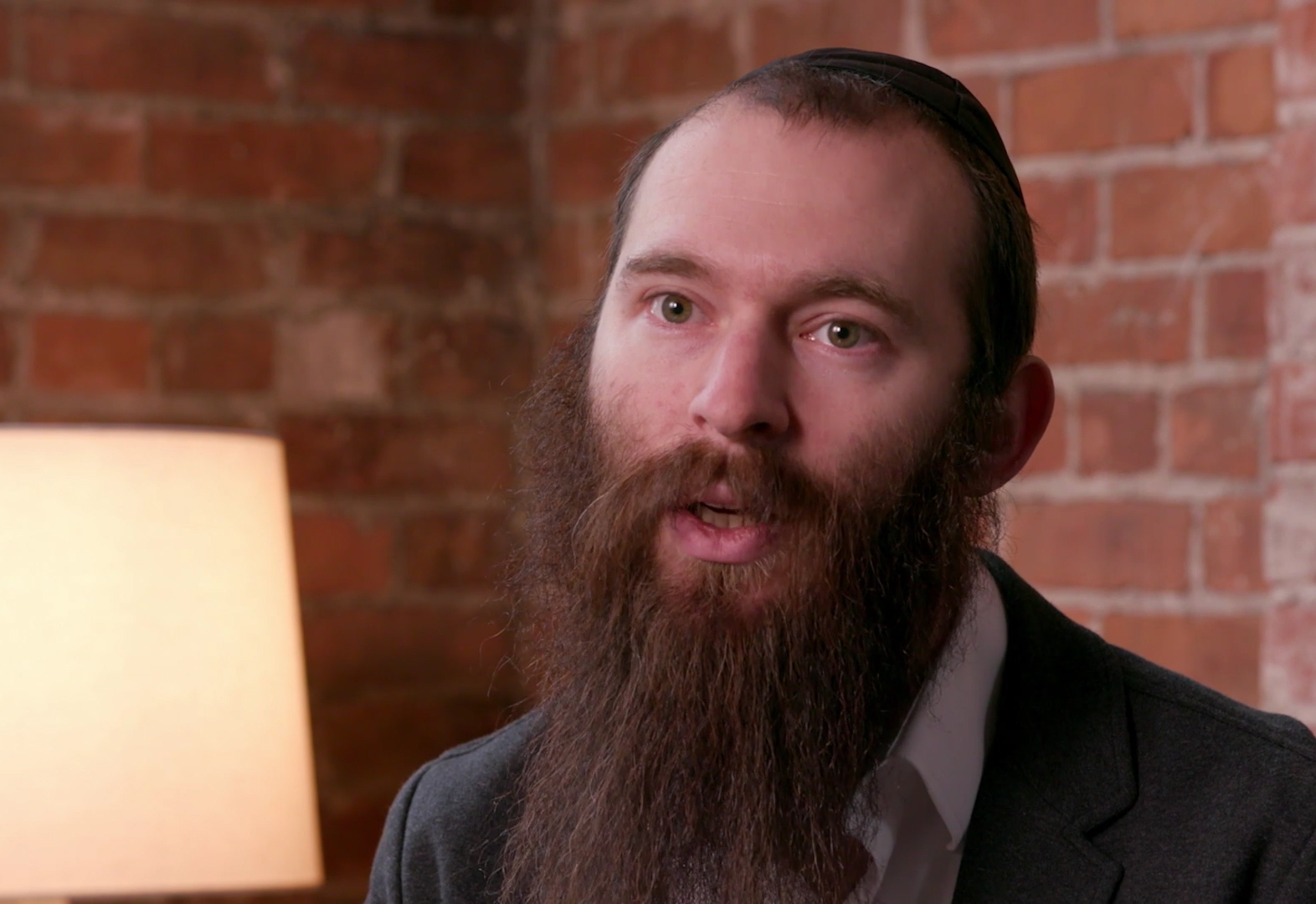### The power of safeTALK suicide awareness training /* <![CDATA[ */ jQuery(document).ready(function(\$){ \$(function(){ var width = \$(window).innerWidth(); var setwidth = 960; var ratio = 0.5625; var height = 540; var link = 'https://vimeo.com/308560662?width=960&height=540'; if(width < setwidth) { height = Math.floor(width * 0.5625); //console.log("device width "+width+", set width "+960+", ratio "+0.5625+", new height "+ height); var new_url = wpvl_paramReplace('width', link, width); var new_url = wpvl_paramReplace('height', new_url, height); \$("a#5c94f3ca3bbf0").attr('href', new_url); //console.log(new_url); } }); }); /* ]]&gt; */

#### watch Yarden's video /* <![CDATA[ */ jQuery(document).ready(function(\$){ \$(function(){ var width = \$(window).innerWidth(); var setwidth = 960; var ratio = 0.5625; var height = 540; var link = 'https://vimeo.com/308560662?width=960&height=540'; if(width < setwidth) { height = Math.floor(width * 0.5625); //console.log("device width "+width+", set width "+960+", ratio "+0.5625+", new height "+ height); var new_url = wpvl_paramReplace('width', link, width); var new_url = wpvl_paramReplace('height', new_url, height); \$("a#5c94f3ca3bc0c").attr('href', new_url); //console.log(new_url); } }); }); /* ]]&gt; */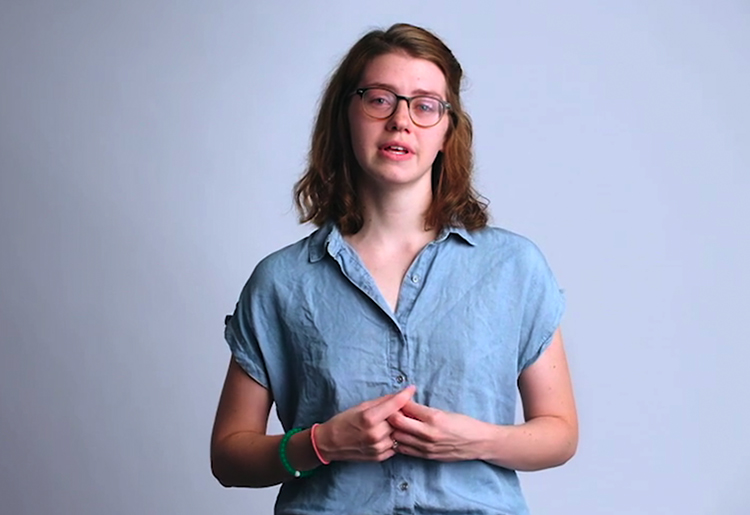### Anxiety, self-harm and recovery /* <![CDATA[ */ jQuery(document).ready(function(\$){ \$(function(){ var width = \$(window).innerWidth(); var setwidth = 960; var ratio = 0.5625; var height = 540; var link = 'https://vimeo.com/295396898?width=960&height=540'; if(width < setwidth) { height = Math.floor(width * 0.5625); //console.log("device width "+width+", set width "+960+", ratio "+0.5625+", new height "+ height); var new_url = wpvl_paramReplace('width', link, width); var new_url = wpvl_paramReplace('height', new_url, height); \$("a#5c94f3ca3bc46").attr('href', new_url); //console.log(new_url); } }); }); /* ]]&gt; */

#### watch Audrey's video /* <![CDATA[ */ jQuery(document).ready(function(\$){ \$(function(){ var width = \$(window).innerWidth(); var setwidth = 960; var ratio = 0.5625; var height = 540; var link = 'https://vimeo.com/295396898?width=960&height=540'; if(width < setwidth) { height = Math.floor(width * 0.5625); //console.log("device width "+width+", set width "+960+", ratio "+0.5625+", new height "+ height); var new_url = wpvl_paramReplace('width', link, width); var new_url = wpvl_paramReplace('height', new_url, height); \$("a#5c94f3ca3bc60").attr('href', new_url); //console.log(new_url); } }); }); /* ]]&gt; */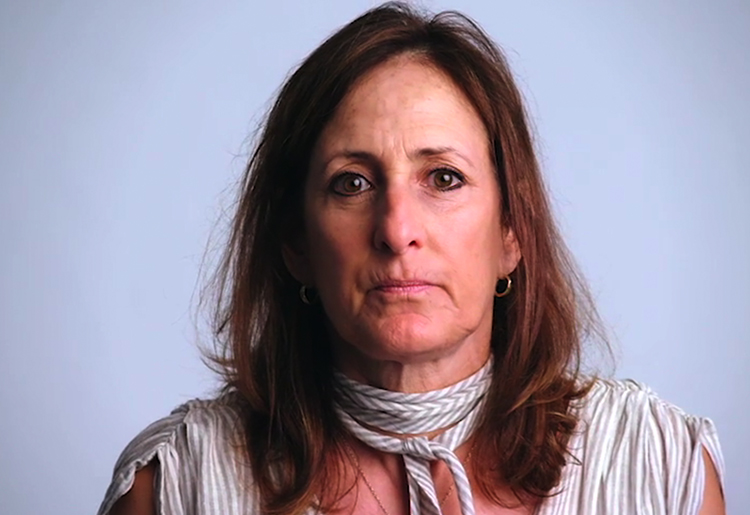### Bipolar disorder: struggle, suicide and the need to speak up /* <![CDATA[ */ jQuery(document).ready(function(\$){ \$(function(){ var width = \$(window).innerWidth(); var setwidth = 960; var ratio = 0.5625; var height = 540; var link = 'https://vimeo.com/295397110?width=960&height=540'; if(width < setwidth) { height = Math.floor(width * 0.5625); //console.log("device width "+width+", set width "+960+", ratio "+0.5625+", new height "+ height); var new_url = wpvl_paramReplace('width', link, width); var new_url = wpvl_paramReplace('height', new_url, height); \$("a#5c94f3ca3bc97").attr('href', new_url); //console.log(new_url); } }); }); /* ]]&gt; */

#### watch Diane's video /* <![CDATA[ */ jQuery(document).ready(function(\$){ \$(function(){ var width = \$(window).innerWidth(); var setwidth = 960; var ratio = 0.5625; var height = 540; var link = 'https://vimeo.com/295397110?width=960&height=540'; if(width < setwidth) { height = Math.floor(width * 0.5625); //console.log("device width "+width+", set width "+960+", ratio "+0.5625+", new height "+ height); var new_url = wpvl_paramReplace('width', link, width); var new_url = wpvl_paramReplace('height', new_url, height); \$("a#5c94f3ca3bcb1").attr('href', new_url); //console.log(new_url); } }); }); /* ]]&gt; */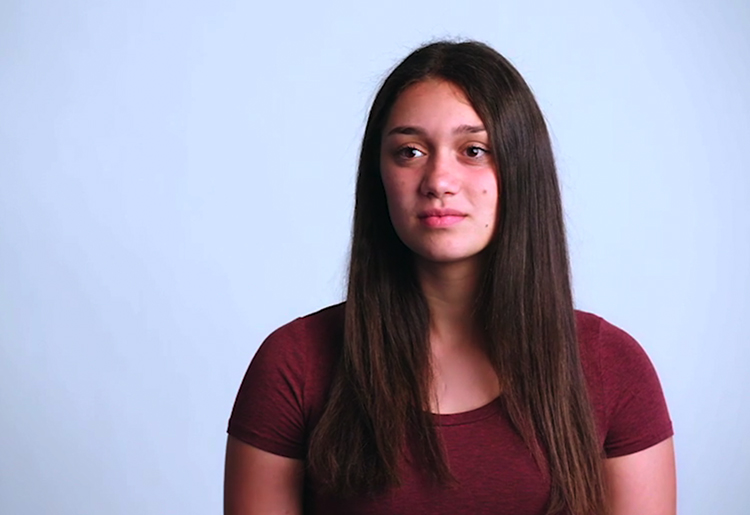### Eating disorders: A family crisis /* <![CDATA[ */ jQuery(document).ready(function(\$){ \$(function(){ var width = \$(window).innerWidth(); var setwidth = 960; var ratio = 0.5625; var height = 540; var link = 'https://vimeo.com/295397338?width=960&height=540'; if(width < setwidth) { height = Math.floor(width * 0.5625); //console.log("device width "+width+", set width "+960+", ratio "+0.5625+", new height "+ height); var new_url = wpvl_paramReplace('width', link, width); var new_url = wpvl_paramReplace('height', new_url, height); \$("a#5c94f3ca3bce5").attr('href', new_url); //console.log(new_url); } }); }); /* ]]&gt; */

#### watch Jamie's video /* <![CDATA[ */ jQuery(document).ready(function(\$){ \$(function(){ var width = \$(window).innerWidth(); var setwidth = 960; var ratio = 0.5625; var height = 540; var link = 'https://vimeo.com/295397338?width=960&height=540'; if(width < setwidth) { height = Math.floor(width * 0.5625); //console.log("device width "+width+", set width "+960+", ratio "+0.5625+", new height "+ height); var new_url = wpvl_paramReplace('width', link, width); var new_url = wpvl_paramReplace('height', new_url, height); \$("a#5c94f3ca3bd08").attr('href', new_url); //console.log(new_url); } }); }); /* ]]&gt; */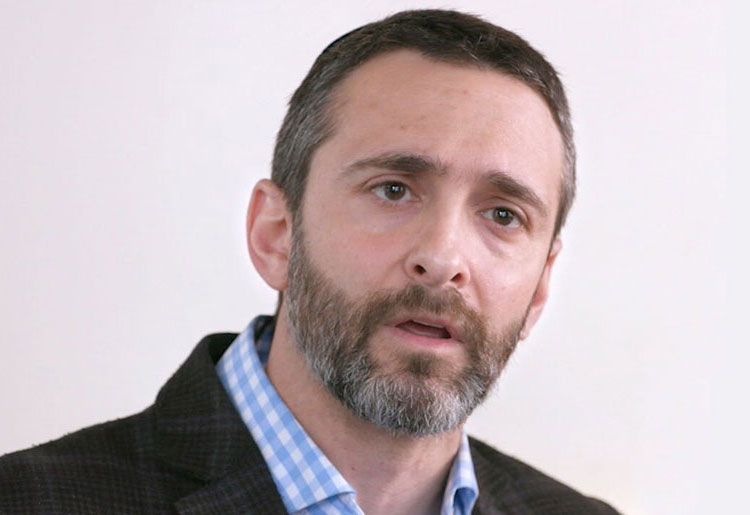### Success does not mean being the best at everything /* <![CDATA[ */ jQuery(document).ready(function(\$){ \$(function(){ var width = \$(window).innerWidth(); var setwidth = 960; var ratio = 0.5625; var height = 540; var link = 'https://vimeo.com/267890415?width=960&height=540'; if(width < setwidth) { height = Math.floor(width * 0.5625); //console.log("device width "+width+", set width "+960+", ratio "+0.5625+", new height "+ height); var new_url = wpvl_paramReplace('width', link, width); var new_url = wpvl_paramReplace('height', new_url, height); \$("a#5c94f3ca3bd3b").attr('href', new_url); //console.log(new_url); } }); }); /* ]]&gt; */

#### watch Azaryah's video /* <![CDATA[ */ jQuery(document).ready(function(\$){ \$(function(){ var width = \$(window).innerWidth(); var setwidth = 960; var ratio = 0.5625; var height = 540; var link = 'https://vimeo.com/267890415?width=960&height=540'; if(width < setwidth) { height = Math.floor(width * 0.5625); //console.log("device width "+width+", set width "+960+", ratio "+0.5625+", new height "+ height); var new_url = wpvl_paramReplace('width', link, width); var new_url = wpvl_paramReplace('height', new_url, height); \$("a#5c94f3ca3bd52").attr('href', new_url); //console.log(new_url); } }); }); /* ]]&gt; */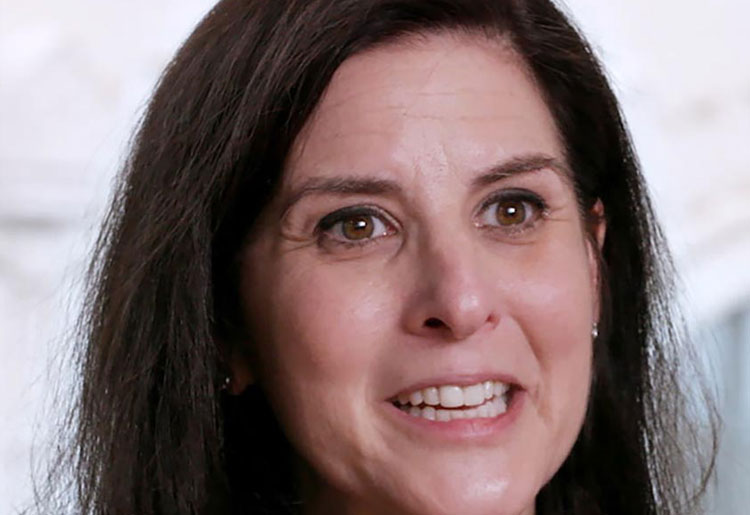### Letting our kids…be kids /* <![CDATA[ */ jQuery(document).ready(function(\$){ \$(function(){ var width = \$(window).innerWidth(); var setwidth = 960; var ratio = 0.5625; var height = 540; var link = 'https://vimeo.com/267890668?width=960&height=540'; if(width < setwidth) { height = Math.floor(width * 0.5625); //console.log("device width "+width+", set width "+960+", ratio "+0.5625+", new height "+ height); var new_url = wpvl_paramReplace('width', link, width); var new_url = wpvl_paramReplace('height', new_url, height); \$("a#5c94f3ca3bd82").attr('href', new_url); //console.log(new_url); } }); }); /* ]]&gt; */

#### watch Julie's video /* <![CDATA[ */ jQuery(document).ready(function(\$){ \$(function(){ var width = \$(window).innerWidth(); var setwidth = 960; var ratio = 0.5625; var height = 540; var link = 'https://vimeo.com/267890668?width=960&height=540'; if(width < setwidth) { height = Math.floor(width * 0.5625); //console.log("device width "+width+", set width "+960+", ratio "+0.5625+", new height "+ height); var new_url = wpvl_paramReplace('width', link, width); var new_url = wpvl_paramReplace('height', new_url, height); \$("a#5c94f3ca3bd99").attr('href', new_url); //console.log(new_url); } }); }); /* ]]&gt; */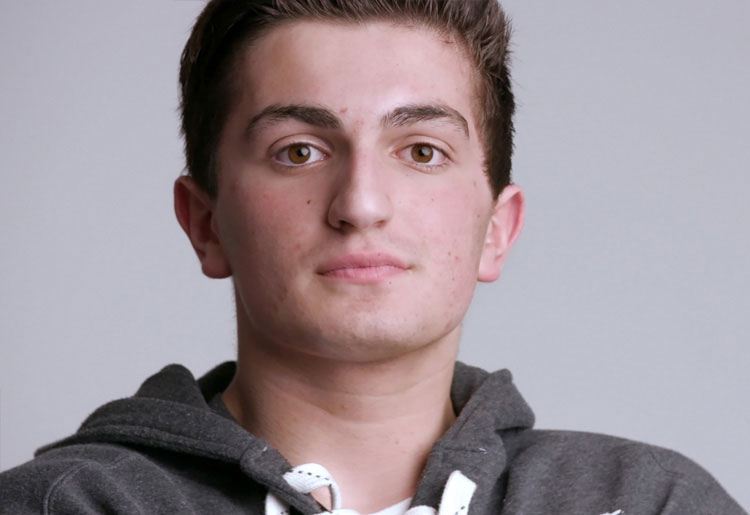### Suicide never impacts just one person /* <![CDATA[ */ jQuery(document).ready(function(\$){ \$(function(){ var width = \$(window).innerWidth(); var setwidth = 960; var ratio = 0.5625; var height = 540; var link = 'https://vimeo.com/267890314?width=960&height=540'; if(width < setwidth) { height = Math.floor(width * 0.5625); //console.log("device width "+width+", set width "+960+", ratio "+0.5625+", new height "+ height); var new_url = wpvl_paramReplace('width', link, width); var new_url = wpvl_paramReplace('height', new_url, height); \$("a#5c94f3ca3bdc8").attr('href', new_url); //console.log(new_url); } }); }); /* ]]&gt; */

#### watch Adam's video /* <![CDATA[ */ jQuery(document).ready(function(\$){ \$(function(){ var width = \$(window).innerWidth(); var setwidth = 960; var ratio = 0.5625; var height = 540; var link = 'https://vimeo.com/267890314?width=960&height=540'; if(width < setwidth) { height = Math.floor(width * 0.5625); //console.log("device width "+width+", set width "+960+", ratio "+0.5625+", new height "+ height); var new_url = wpvl_paramReplace('width', link, width); var new_url = wpvl_paramReplace('height', new_url, height); \$("a#5c94f3ca3bdee").attr('href', new_url); //console.log(new_url); } }); }); /* ]]&gt; */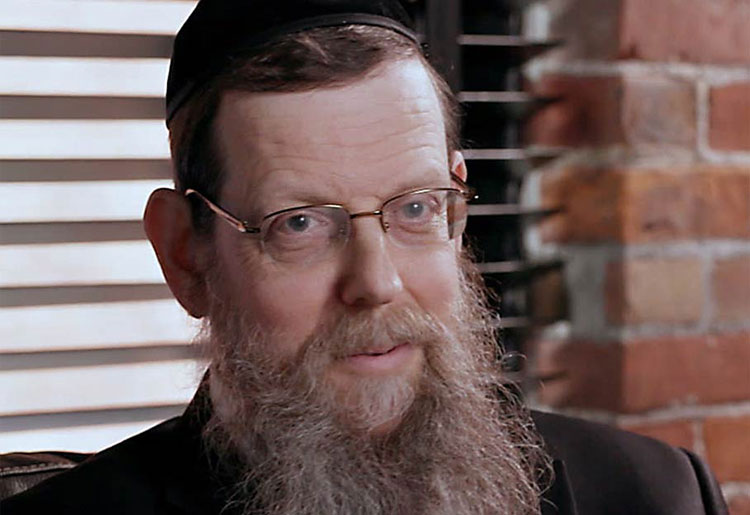### Mental health issues don’t discriminate /* <![CDATA[ */ jQuery(document).ready(function(\$){ \$(function(){ var width = \$(window).innerWidth(); var setwidth = 960; var ratio = 0.5625; var height = 540; var link = 'https://vimeo.com/267890824?width=960&height=540'; if(width < setwidth) { height = Math.floor(width * 0.5625); //console.log("device width "+width+", set width "+960+", ratio "+0.5625+", new height "+ height); var new_url = wpvl_paramReplace('width', link, width); var new_url = wpvl_paramReplace('height', new_url, height); \$("a#5c94f3ca3be1f").attr('href', new_url); //console.log(new_url); } }); }); /* ]]&gt; */

#### watch Rabbi Zimmerman's video /* <![CDATA[ */ jQuery(document).ready(function(\$){ \$(function(){ var width = \$(window).innerWidth(); var setwidth = 960; var ratio = 0.5625; var height = 540; var link = 'https://vimeo.com/267890824?width=960&height=540'; if(width < setwidth) { height = Math.floor(width * 0.5625); //console.log("device width "+width+", set width "+960+", ratio "+0.5625+", new height "+ height); var new_url = wpvl_paramReplace('width', link, width); var new_url = wpvl_paramReplace('height', new_url, height); \$("a#5c94f3ca3be34").attr('href', new_url); //console.log(new_url); } }); }); /* ]]&gt; */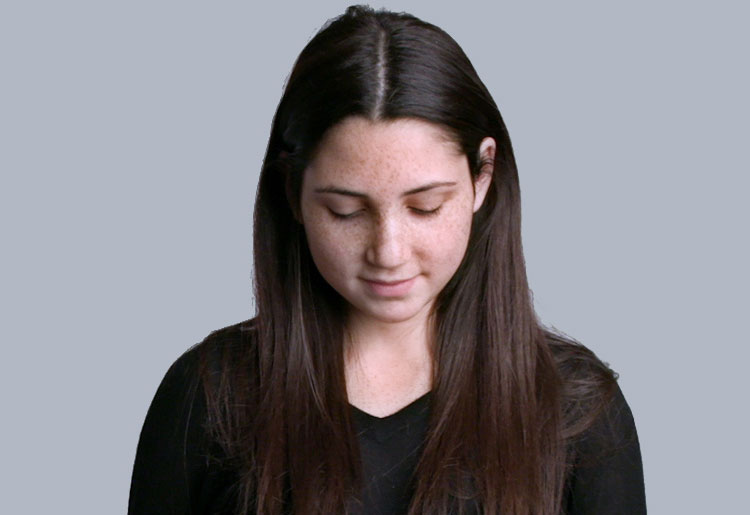### Depression, eating disorders and recovery /* <![CDATA[ */ jQuery(document).ready(function(\$){ \$(function(){ var width = \$(window).innerWidth(); var setwidth = 960; var ratio = 0.5625; var height = 540; var link = 'https://vimeo.com/269235214?width=960&height=540'; if(width < setwidth) { height = Math.floor(width * 0.5625); //console.log("device width "+width+", set width "+960+", ratio "+0.5625+", new height "+ height); var new_url = wpvl_paramReplace('width', link, width); var new_url = wpvl_paramReplace('height', new_url, height); \$("a#5c94f3ca3be5b").attr('href', new_url); //console.log(new_url); } }); }); /* ]]&gt; */

#### watch Stella's video /* <![CDATA[ */ jQuery(document).ready(function(\$){ \$(function(){ var width = \$(window).innerWidth(); var setwidth = 960; var ratio = 0.5625; var height = 540; var link = 'https://vimeo.com/269235214?width=960&height=540'; if(width < setwidth) { height = Math.floor(width * 0.5625); //console.log("device width "+width+", set width "+960+", ratio "+0.5625+", new height "+ height); var new_url = wpvl_paramReplace('width', link, width); var new_url = wpvl_paramReplace('height', new_url, height); \$("a#5c94f3ca3be6e").attr('href', new_url); //console.log(new_url); } }); }); /* ]]&gt; */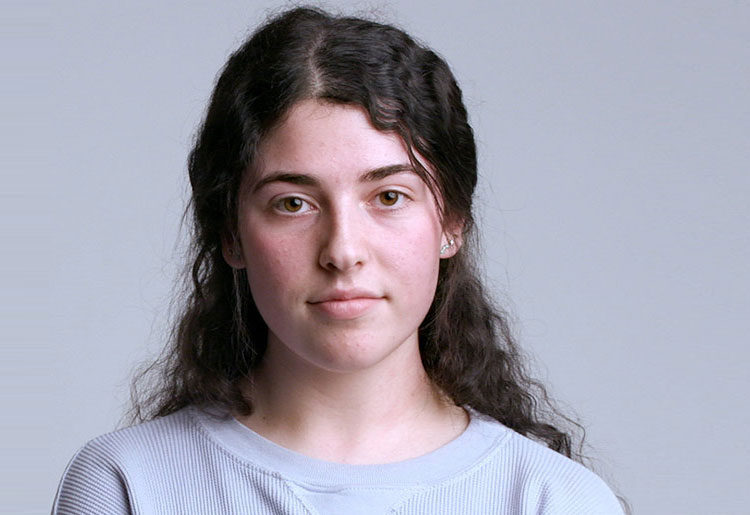### Speaking up is a sign of strength /* <![CDATA[ */ jQuery(document).ready(function(\$){ \$(function(){ var width = \$(window).innerWidth(); var setwidth = 960; var ratio = 0.5625; var height = 540; var link = 'https://vimeo.com/267890533?width=960&height=540'; if(width < setwidth) { height = Math.floor(width * 0.5625); //console.log("device width "+width+", set width "+960+", ratio "+0.5625+", new height "+ height); var new_url = wpvl_paramReplace('width', link, width); var new_url = wpvl_paramReplace('height', new_url, height); \$("a#5c94f3ca3be95").attr('href', new_url); //console.log(new_url); } }); }); /* ]]&gt; */

#### watch Hannah's video /* <![CDATA[ */ jQuery(document).ready(function(\$){ \$(function(){ var width = \$(window).innerWidth(); var setwidth = 960; var ratio = 0.5625; var height = 540; var link = 'https://vimeo.com/267890533?width=960&height=540'; if(width < setwidth) { height = Math.floor(width * 0.5625); //console.log("device width "+width+", set width "+960+", ratio "+0.5625+", new height "+ height); var new_url = wpvl_paramReplace('width', link, width); var new_url = wpvl_paramReplace('height', new_url, height); \$("a#5c94f3ca3bea7").attr('href', new_url); //console.log(new_url); } }); }); /* ]]&gt; */